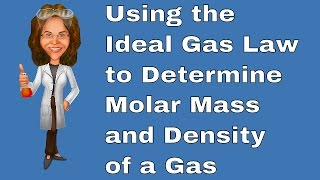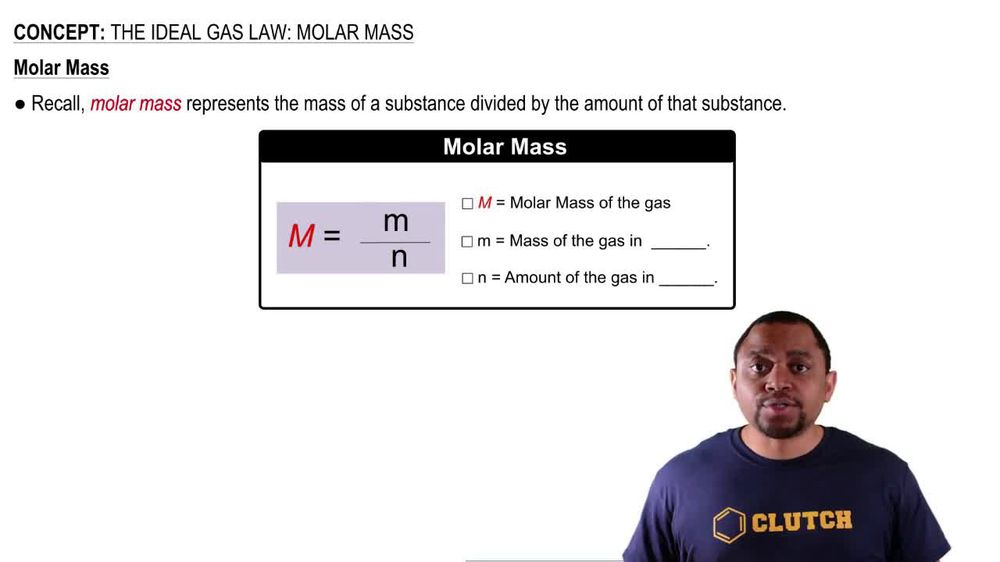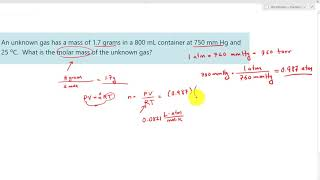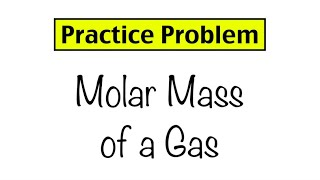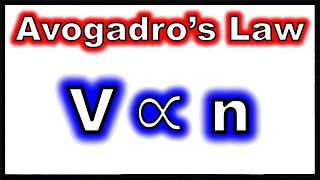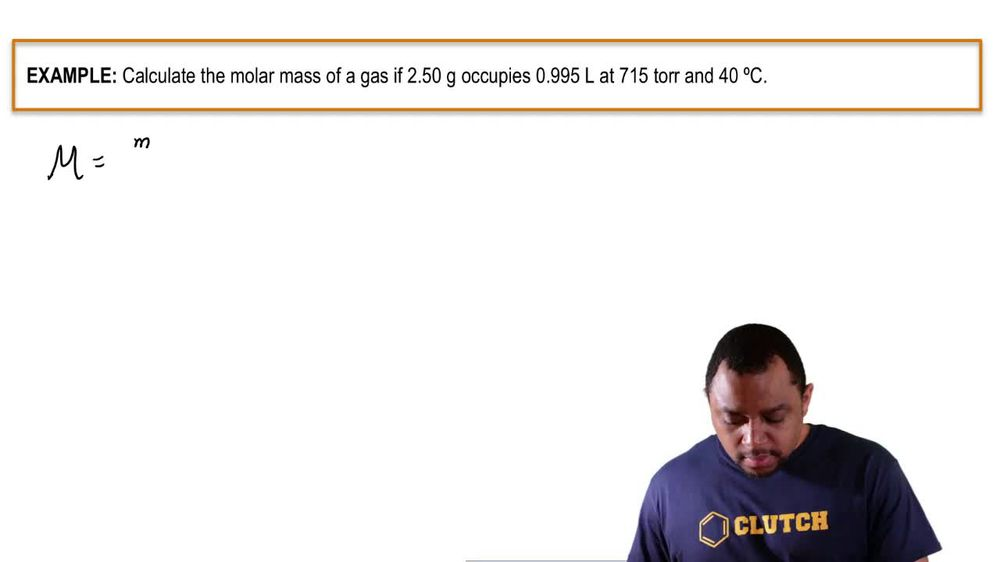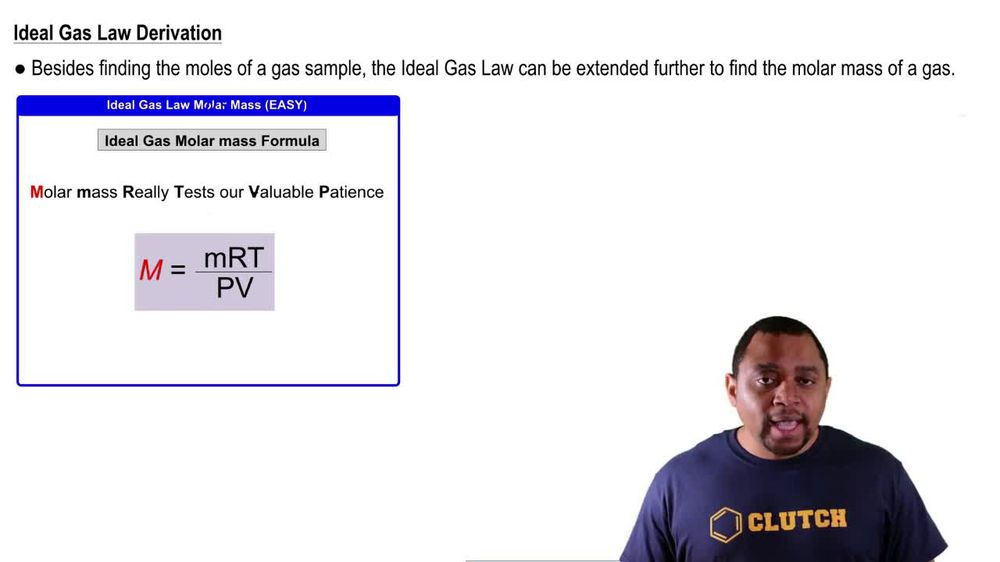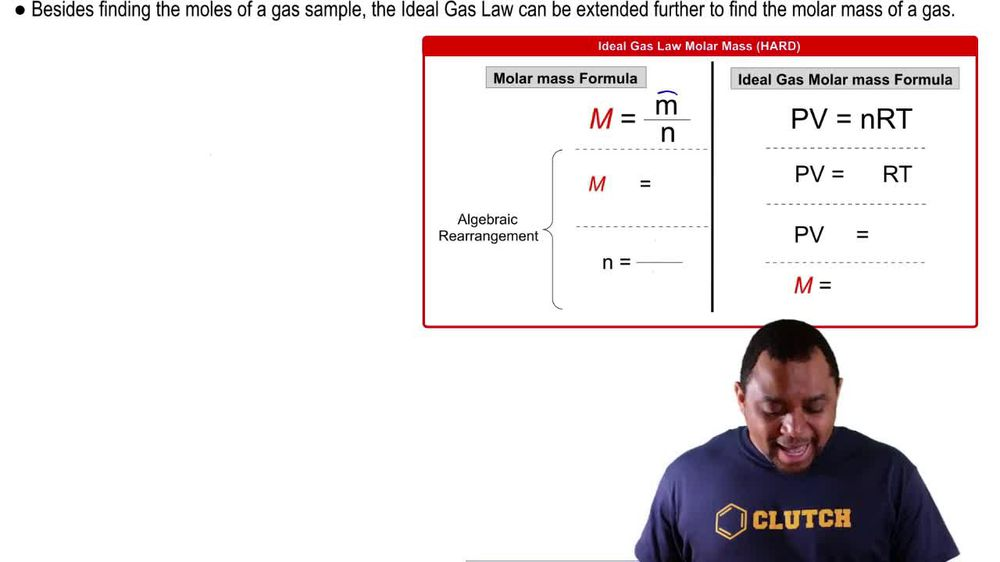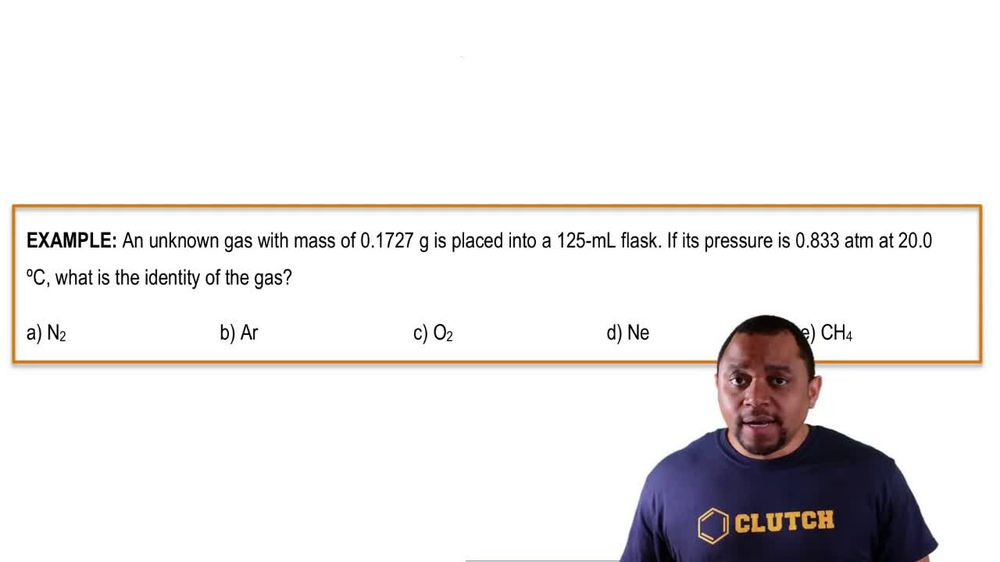Start typing, then use the up and down arrows to select an option from the list.
1. 7. Gases2. The Ideal Gas Law: Molar Mass# The Ideal Gas Law: Molar Mass

by Jules Bruno
157 views
0
up to this point. We've used the ideal gas law to help us isolate pressure volume, moles or temperature. But now we're gonna be faced with a new but old topic. The idea of Moeller Mass. Now recall Moeller Mass represents the mass of a substance divided by the amount of that substance. If we take a look here, we're going to say that this capital M represents the molar mass of the gas and lower case M here equals the mass of the gas Ingram's and And we know from the ideal gas law equals the amount off the gas in moles. So more mass equals grams promote. Now that we remember what more Masses, we're gonna take a look at how we can use the ideal gas law to find the more mass of a gas. So for now, click on the next video and let's take a look. An example question